Mathematics
Easy

Question

# Simplify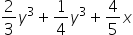##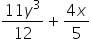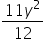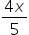xyHint:

## The correct answer is:### The given expression is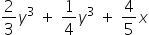There are two variables in the above expression. The variables are: ‘x’ and ‘y’. There are three terms in the above expression. We have to perform the operations between them and simplify the expression.If we see, first and second term contains the same variable raised to same power 3. Such terms are called “like terms.” And the terms with different variables are called “unlike terms.”The third term has different variable.For like terms, we can perform the operations between their coefficients by keeping the variable constant. It means we can treat each term as a constant and perform operations like normal operations on constants.For the above expression, we can perform an operation between the first term and second term as both the terms as they have the same variable ‘y3'. We will keep ‘y3’ constant and perform addition between the coefficients.Let’s see the expression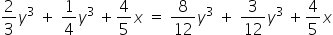To make the denominators of the first and second terms the same, we multiply and divide the first term by the denominator of the second term and vice versa.Solving further we get,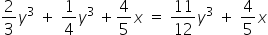So this is the final value.

We have to be careful about the power of variable to. The variables with same power can operate. If we have same variable but different power no further operation is possible.

### Related Questions to study#### With Turito Foundation.#### Get an Expert Advice From Turito.